Question

# A linear dc machine has a magnetic flux density of 0.5T directed into the page, a...

A linear dc machine has a magnetic flux density of 0.5T directed into the page, a resistance of 0.25Ω, a bar length of 1m, and a battery voltage of 100V.

a) Create a mathlab script that plots the following (x vs y):

Force (0:5:100N) vs Velocity#### Earn Coins

Coins can be redeemed for fabulous gifts.

Similar Homework Help Questions
• ### Question5 (5 points) A linear motor composed of conducting rod (the rotor) and permanent magnetic stator that provides a uniform magnetic flux density of 0.8 tesla in the shown direction. The rai...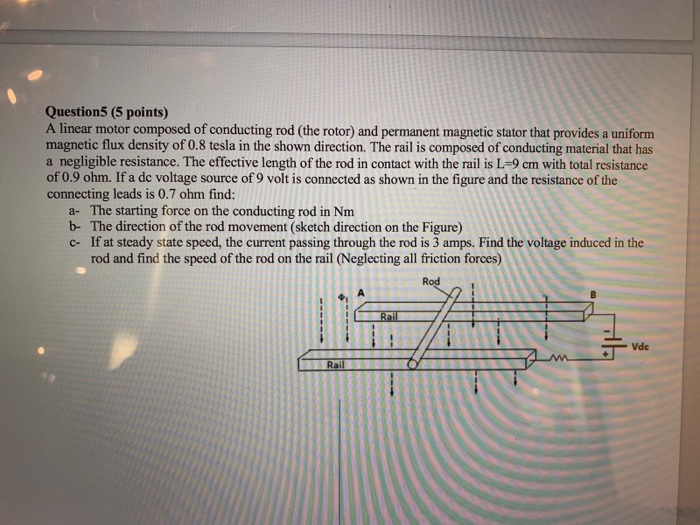Question5 (5 points) A linear motor composed of conducting rod (the rotor) and permanent magnetic stator that provides a uniform magnetic flux density of 0.8 tesla in the shown direction. The rail is composed of conducting material that has a negligible resistance. The effective length of the rod in contact with the rail is L-9 cm with total resistance of 0.9 ohm. If a dc voltage source of 9 volt is connected as shown in the figure and the resistance...

• ### 8.) (30 points) A rectangular frame of conducting wire has negligible resistance and a width 1.0 m is placed in a magnetic field (directed into the page) of 2.0 T, as shown in the figure A m...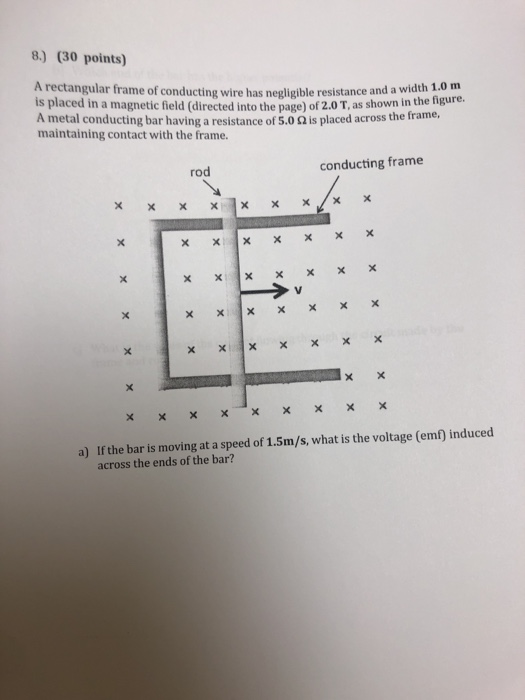8.) (30 points) A rectangular frame of conducting wire has negligible resistance and a width 1.0 m is placed in a magnetic field (directed into the page) of 2.0 T, as shown in the figure A metal conducting bar having a resistance of 5.0Ω is placed across the frame, maintaining contact with the frame. conducting frame rod If the bar is moving at a speed of 1.5m/s, what is the voltage (em) induced across the ends of the bar? a)...

• ### A DC generator can be built as shown in the figure below. The bar, load (R),...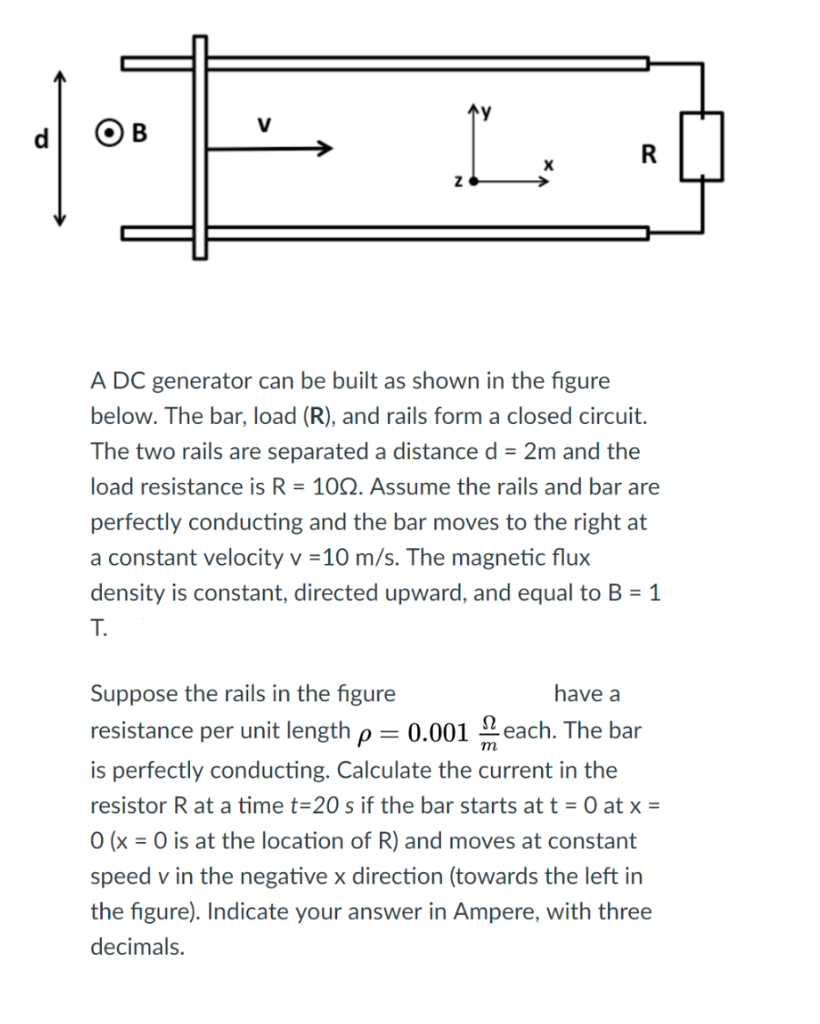A DC generator can be built as shown in the figure below. The bar, load (R), and rails form a closed circuit. The two rails are separated a distance d- 2m and the load resistance is R- 10Q. Assume the rails and bar are perfectly conducting and the bar moves to the right at a constant velocity v-10 m/s. The magnetic flux density is constant, directed upward, and equal to B-1 T. Suppose the rails in the figure resistance per...

• ### solve part c Hìnt: The following identities will be useful: LWI) PVI sin e sin 2ot...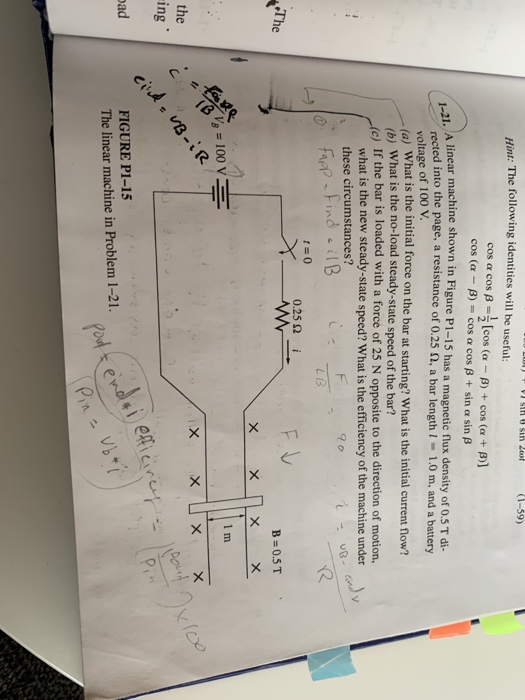solve part c Hìnt: The following identities will be useful: LWI) PVI sin e sin 2ot (1-59) cos a cos B = [cos (a - B) + cos (a + B)] cos (a - B) - cos a cos B + sin a sin B 1-21. A linear machine shown in Figure P1-15 has a magnetic flux density of 0.5 T di- rected into the page, a resistance of 0.25 N, a bar length I=- 1.0 m, and a battery...

• ### Question 7 The conducting rod shown in the figure has length L and is being pulled along horlizontal, frictionless, conducting rails at a constant metal strip. A uniform magnetic field, directed...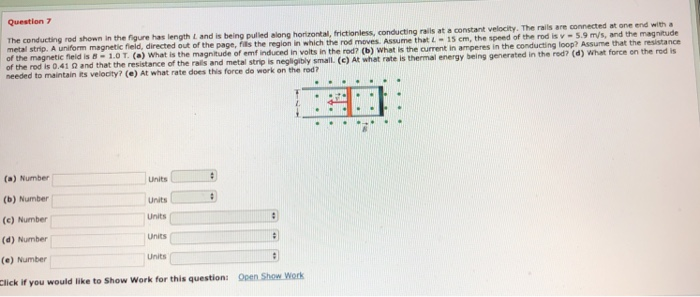Question 7 The conducting rod shown in the figure has length L and is being pulled along horlizontal, frictionless, conducting rails at a constant metal strip. A uniform magnetic field, directed of the magnetic fieid is 8-1.0 T. (a) What is the magnitude Assume that L15 cm, the speed of the rod is v -5.9 m/s, and the magnitude of emf induced in voits in the rod? (b) What is the current in amperes in the conducting loop? Assume that...

• ### A rectangular wire loop located in the plane of the page has a width L, and its length. X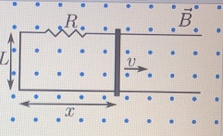A rectangular wire loop located in the plane of the page has a width L, and its length. X. is determined by the position a movable rail that forms the fourth side of the rectangle, as shown. The total electrical resistance of the wire loop is R, and an externally applied magnetic field, B, is directed out of the page. The rail is moving towards the right with speed v. Assume that the x direction is towards the right of...

• ### of an electromagnetic wave propagating in a vacuum is 65 x 10 Hz. See the table for problem 1 1) To what part of the electromagnetic spectrum does this wave a) blue light 2) The electromag a)per...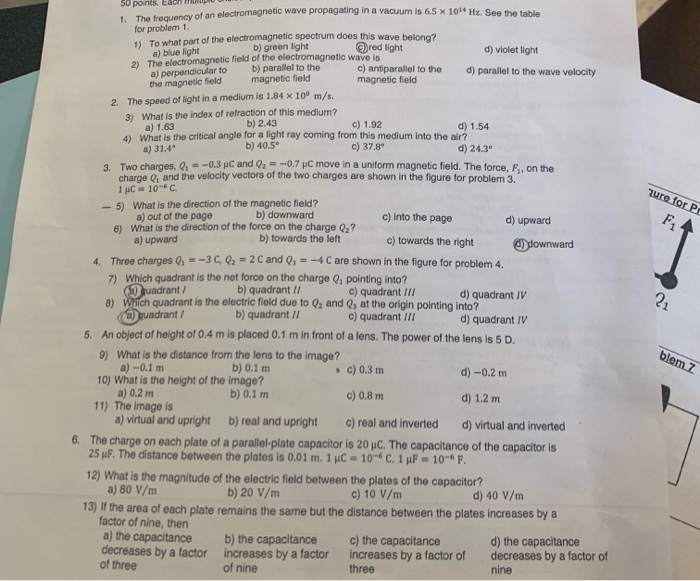of an electromagnetic wave propagating in a vacuum is 65 x 10 Hz. See the table for problem 1 1) To what part of the electromagnetic spectrum does this wave a) blue light 2) The electromag a)perpendicular to b) parallel to the the magnetic field b) green light netic field of the electromagnetic wave is d) violet light c) antiparallel to the d) paralel to the wave velocity magnetic field magnetic field The speed of light in a medium is...